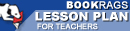Lesson Plans

Longitude: The True Story of a Lone Genius Who Solved the Greatest Scientific Problem of His Time Multiple Choice Test Questions

This set of Lesson Plans consists of approximately 126 pages of tests, essay questions, lessons, and other teaching materials.
 View a FREE sampleChapter 1: Imaginary Lines

1. What toy does the narrator play with at the beginning of Chapter 1: Imaginary Lines?
(a) A spinning top.
(c) A yo-yo.
(d) A globe.

2. The difference between longitude and latitude is that latitude lines _____________ each other.
(a) Converge with.
(b) Intersect.
(c) Parallel.
(d) Bisect.

3. In AD 150, who plotted lines of longitude and latitude?
(a) Ptolemy.
(b) Moses.
(c) Newton.
(d) Galileo.

4. In the earliest lines of latitude, what marked the zero-degree line?
(a) The tropic of cancer.
(b) The north pole.
(c) The equator.
(d) The prime meridian.

5. The placement of the prime meridian was a ____________ decision.
(a) Historical.
(b) Political.
(c) Economic.
(d) Social.

6. What is the main difference in measuring longitude and latitude?
(a) Latitude is measured by time.
(b) Latitude is measured in degrees and longitude is not.
(c) Longitude is measured by time.
(d) Longitude is measured by the sun.

7. When measuring longitude, each hour of time difference between the ship and starting point marks a progress of how many degrees of longitude?
(a) 5.
(b) 25.
(c) 15.
(d) 30.

 This section contains 4,626 words (approx. 16 pages at 300 words per page) View a FREE sample